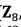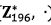107 年 - 107 國立中山大學_碩士班招生考試_資工系(甲組)：離散數學#105787

【非選題】
1.
1. Find the coefficient of x7 in each of the following formulas.

【題組】(a) ((x3-3x)(1-x)5)+3x7+5.

【非選題】
2.【題組】(b) -2/((x-1)(x-2).

【非選題】
3.
2. Consider the counting of the number of onto functions H: A →B where IA I≥ IBI.

【題組】

(a) Find the exponential generating function for the above counting such that the  coefficient ofis the answer of the above counting.

【非選題】
4.【題組】(b) Apply the above exponential generating function to find the answer of the above counting where |A| = 10 and |B| = 3.

【非選題】
5.
3. Let Σ= [0, 1] be an alphabet and A = [1, 00, 10] be a subset of Σ*.

【題組】

(a) For each integer n ≥ 1, let an be the number of strings in A* of length n. Find and solve a recurrence relation for.

【非選題】
6.【題組】

(b) For each integer n z 1, let bn be the number of strings in A* which are of length n and exactly divided by 2 when we regard each of the strings as a binary number. Find.

【非選題】
7.

4. Consider the additive group (,+).

【題組】 (a) What is the order of 6? Why?

【非選題】
8.【題組】(b) Find all generators of the group.

【非選題】
9.

5. Consider the multiplicative group ().

【題組】 (a) What is the order of the group? Why?

【非選題】
10.【題組】(b) Find the inverse of 25.

【非選題】
11.
6. Let (ZxZ,⊕) be the group with (a, b)⊕(c, d) = (atc+2, 6+d-2) for any (a, b), (c, d) ∈ ZxZ where a+c+2 and b+d-2 are computed using ordinary addition and subtraction in Z.

【題組】(a)3(4,5)=(4,5)⊕(4,5)⊕(4,5)=?

【非選題】
12.【題組】(b) What is the identity of the group?

【非選題】
13.【題組】(c) What is the inverse of (a, b)?

【非選題】
14.【題組】(d) [8%] Let (G, +) be an additive group and let h: ZxZ → G be a group homomorphism where h(3, 2) = u and h(-2,6) = v. Please express h(18, 22) in terms of u and v.